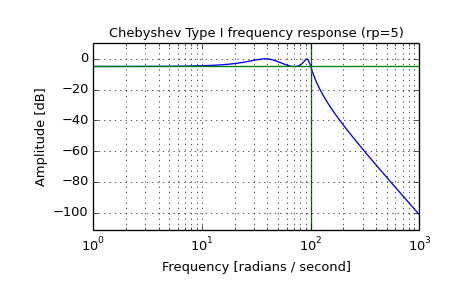scipy.signal.cheby1¶

scipy.signal.cheby1(N, rp, Wn, btype='low', analog=False, output='ba')[source]

Chebyshev type I digital and analog filter design.

Design an Nth order digital or analog Chebyshev type I filter and return the filter coefficients.

Parameters: N : int The order of the filter. rp : float The maximum ripple allowed below unity gain in the passband. Specified in decibels, as a positive number. Wn : array_like A scalar or length-2 sequence giving the critical frequencies. For Type I filters, this is the point in the transition band at which the gain first drops below -rp. For digital filters, Wn is normalized from 0 to 1, where 1 is the Nyquist frequency, pi radians/sample. (Wn is thus in half-cycles / sample.) For analog filters, Wn is an angular frequency (e.g. rad/s). btype : {‘lowpass’, ‘highpass’, ‘bandpass’, ‘bandstop’}, optional The type of filter. Default is ‘lowpass’. analog : bool, optional When True, return an analog filter, otherwise a digital filter is returned. output : {‘ba’, ‘zpk’, ‘sos’}, optional Type of output: numerator/denominator (‘ba’), pole-zero (‘zpk’), or second-order sections (‘sos’). Default is ‘ba’. b, a : ndarray, ndarray Numerator (b) and denominator (a) polynomials of the IIR filter. Only returned if output='ba'. z, p, k : ndarray, ndarray, float Zeros, poles, and system gain of the IIR filter transfer function. Only returned if output='zpk'. sos : ndarray Second-order sections representation of the IIR filter. Only returned if output=='sos'.

cheb1ord

Notes

The Chebyshev type I filter maximizes the rate of cutoff between the frequency response’s passband and stopband, at the expense of ripple in the passband and increased ringing in the step response.

Type I filters roll off faster than Type II (cheby2), but Type II filters do not have any ripple in the passband.

The equiripple passband has N maxima or minima (for example, a 5th-order filter has 3 maxima and 2 minima). Consequently, the DC gain is unity for odd-order filters, or -rp dB for even-order filters.

The 'sos' output parameter was added in 0.16.0.

Examples

Plot the filter’s frequency response, showing the critical points:

>>> from scipy import signal
>>> import matplotlib.pyplot as plt

>>> b, a = signal.cheby1(4, 5, 100, 'low', analog=True)
>>> w, h = signal.freqs(b, a)
>>> plt.semilogx(w, 20 * np.log10(abs(h)))
>>> plt.title('Chebyshev Type I frequency response (rp=5)')
>>> plt.ylabel('Amplitude [dB]')
>>> plt.margins(0, 0.1)
>>> plt.grid(which='both', axis='both')
>>> plt.axvline(100, color='green') # cutoff frequency
>>> plt.axhline(-5, color='green') # rp
>>> plt.show()Previous topic

scipy.signal.buttord

Next topic

scipy.signal.cheb1ord